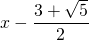# Solution assignment 06 Root equations

### Assignment 6

Solve: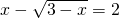### Solution

We can first try to write the conditions which must be met for which this equation is defined. We can also begin solving the equation and check afterwards whether solutions satisfy the equation. We now choose the second approach.
We write: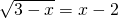We square both sides of the equation: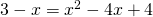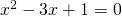This quadratic equation has the following solutions (by applying the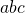-formula):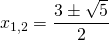or: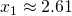or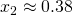Now we check whether these solutions satisfy the original equation. The first solution does satisfy, the second does not: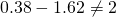Thus the solution of the equation is: